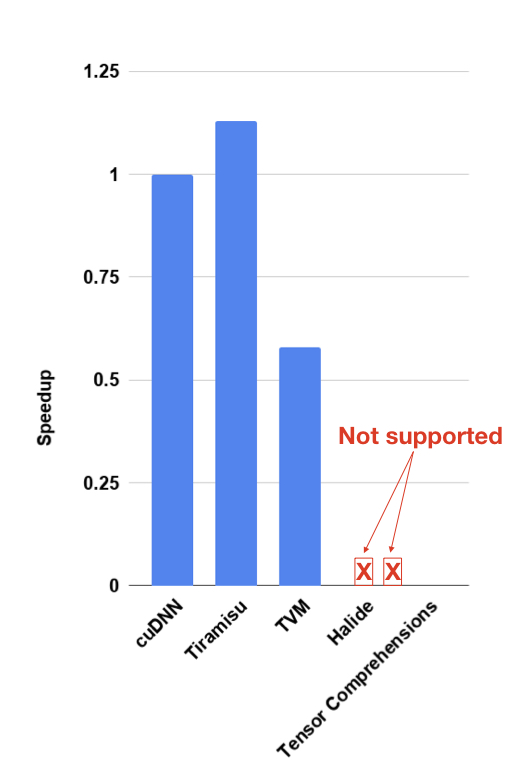# Difference between Tiramisu, TVM and Halide

Tiramisu has fundamental differences compared to TVM and Halide. This post shows some of them.

## Tiramisu is a Polyhedral Compiler

The first difference is that Tiramisu uses the extended polyhedral model internaly while TVM and Halide use intervals. This allows Tiramisu to naturally express many code patterns and transformations that are difficult to express in TVM and Halide. For example:

#### Tiramisu can Optimize RNNs

Tiramisu can optimize RNNs (recurrent neural networks) and generate code that is 2x faster than TVM. This is mainly because Tiramisu can apply a transformation known as iteration space skewing while TVM does not. This transformation allows Tiramisu to increase GPU occupancy and parallelize multi-layer LSTMs by exploiting wave-front parallelism. This optimization is crucial to match highly optimized libraries such as cuDNN and get the best performance out of architectures such as GPUs as described here (check step 3).

The following figure shows a comparison between Tiramisu, cuDNN, TVM, Halide and Tensor Comprehensions on a dynamic LSTM for GPUs. In the next section we will explain why Halide does not support dynamic LSTMs (and programs with cyclic-dataflow graph in general).LSTM GPU

#### Tiramisu can Express Programs with Cyclic DataFlow Graphs

While Halide can implement a simple version of LSTMs (Long Short Term Memory), it is limited to LSTMs where the number of recurrence steps (i.e., cells) is known at compile time. Supporting a dynamic number of recurrence steps is important in case the number of steps is unknown at compile time (which is usually the case in graph and tree LSTMs for example). The inability to express RNNs stems from the fact that Halide has a restricted language that prevents the user from writing a program that has a cycle in its data flow graph since it cannot guarantee the correctness of loop optimizations in that case. Unlike Halide, Tiramisu performs polyhedral dependence analysis and uses transformation legality checks to guarantee the correctness of code transformations. This gives the compiler more flexibility and removes an important restriction on the Tiramisu language.

#### Tiramisu can Represent Non-rectangular Loops Naturally

TVM and Halide use intervals to represent the iteration space of loops (loop iterator ranges). It uses interval arithmetic to transform those intervals. Because they use intervals and interval arithmetic they can only represent loops that are rectangular (i.e., do not have a conditional where the condition depends on one of the loop iterators).

The following code is an example of code that Halide does not support as it creates a non-rectangular iteration space. This code is a simplified version of the following bug report in the Halide repo.

``````//Halide github issue #2373
for x in 0 … N
for y in 0 … N
for r in 0 … 32
if (x >= r)
out(x, y, r) = in(x - r, y);
``````

#### Tiramisu can Generate Efficient Distributed Code

Tiramisu uses the polyhedral model to compute the communication sets when generating code for distributed architectures. This allows Tiramisu to compute exactly the amount of data that needs to be sent/received by each node. The Tiramisu paper has a performance comparison between distributed code generated by Tiramisu and that generated by halide.

#### Tiramisu Can Apply Any Affine Transformation

Tiramisu, being polyhedral, can support any affine transformation on loop nests and data accesses. No matter how the affine transformation is complicated, Tiramisu can do it. TVM and Halide do not support all the class of affine transformations.

## Tiramisu Supports Sparse DNNs

Tiramisu is currently the only DNN compiler that supports sparse DNNs.